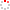#CodeStepByStep## Sieve

Language/Type: C# arrays interactive programs

Write a complete console program named `Sieve` that uses the "Sieve of Eratosthenes" algorithm to print a list of prime numbers between 2 and a given maximum. You will represent the numbers using an array.

In the third century B.C., the Greek astronomer Eratosthenes developed an algorithm for finding all the prime numbers up to some upper limit N. To apply the algorithm, you start by writing down a list of the integers between 2 and N. For example, if N is 10, you would write down the following list:

```2 3 4 5 6 7 8 9 10
```

You then underline the first number in the list and cross off every multiple of that number. Thus, after executing the first step of the algorithm, you will underline 2 and cross off every multiple of 2:

```2 3 4 5 6 7 8 9 10
```

From here, you simply repeat the process: underline the first number in the list that is neither crossed nor underlined, and then cross off its multiples. Eventually, every number in the list will either be underlined or crossed out, as shown below. The underlined numbers are prime.

```2 3 4 5 6 7 8 9 10
```

Your program should prompt the user to enter a max value N, and then perform the Sieve of Eratosthenes algorithm on the range of numbers 2 through N inclusive. You may assume that the user types a number that is at least 2. Here is an example output from one run of your program, with user input shown like this:

```Max value N? 100
Primes: 2 3 5 7 11 13 17 19 23 29 31 37 41 43 47 53 59 61 67 71 73 79 83 89 97
```

You must use an array in your solution to this problem. Part of your task in this problem is figuring out how to use an array to represent the list of numbers and how to remember which ones are prime, which ones are "underlined" vs "crossed off," and so on.

Type your C# solution code here:

This exercise asks for a complete program. This should essentially be contents you could put into a file and run outside of CodeStepByStep.